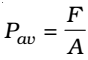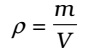Fluids are those substances which can flow when an external force is applied to it. Liquids and gases are fluids. Fluids do not have finite shape but take the shape of the containing vessel. The total normal force exerted by the liquid at rest on a given surface is called thrust of liquid. The SI unit of thrust is Newton.

In fluid mechanics the following properties of fluid would be considered:

1. When the fluid is at rest – hydrostatics
2. When the fluid is in motion – hydrodynamics

## Pressure:

The thrust experienced per unit area of the surface of a liquid at rest is called pressure.In principle, the piston area can be made arbitrarily small. The pressure is then defined in a limiting sense asPressure is a scalar quantity. Its dimensions are [ML-1T-2]. The SI unit of pressure is N m-2.

Another quantity, that is indispensable in describing fluids, is the density ρ. For a fluid of mass m occupying volume V,The dimensions of density are [ML-3]. Its SI unit is kg m-3. It is a positive scalar quantity. A liquid is largely incompressible and its density is, therefore, nearly constant at all pressures. Gases, on the other hand, exhibit a large variation in densities with pressure.

### Pressure exerted by a liquid column

P = hρg

Where, h = height of liquid column,

ρ = density of liquid and

g = acceleration due to gravity

Mean pressure on the walls of a vessel containing liquid up to height h is (h ρ g / 2).

## Buoyancy

When a body is partially or fully immersed in a fluid an upward force acts on it, which is called buoyant force or simply buoyancy.
The buoyant force acts at the centre of gravity of the liquid displaced] by the immersed part of the body and this point is called the centre buoyancy.

## Archimede’s Principle

When a body is partially or fully immersed in a liquid, it loses some of its weight and it is equal to the weight of the liquid displaced by the immersed part of the body. If T is the observed weight of a body of density σ when it is fully immersed in a liquid of density p, then real weight of the body